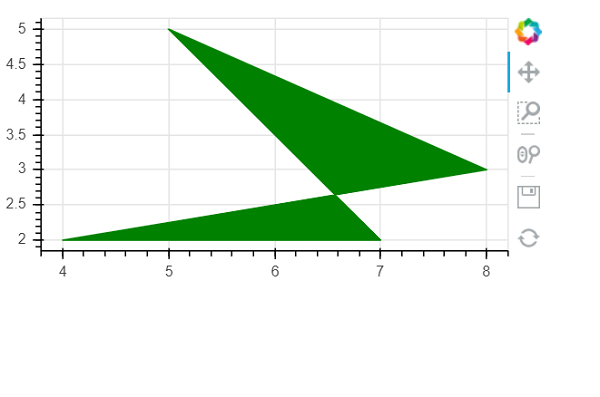# How can Bokeh be used to generate patch plot in Python?

Bokeh is a Python package that helps in data visualization. It is an open source project. Bokeh renders its plot using HTML and JavaScript. This indicates that it is useful while working with web-based dashboards.

Matplotlib and Seaborn produce static plots, whereas Bokeh produces interactive plots. This means when the user interacts with these plots, they change accordingly.

Plots can be embedded as output of Flask or Django enabled web applications. Jupyter notebook can also be used to render these plots.

Dependencies of Bokeh −

Numpy
Pillow
Jinja2
Packaging
Pyyaml
Six
Python−dateutil

Installation of Bokeh on Windows command prompt

pip3 install bokeh


Installation of Bokeh on Anaconda prompt

conda install bokeh

Let us see an example −

## Example

from bokeh.plotting import figure, output_file, show
p = figure(plot_width = 400, plot_height = 200)
p.patch(x = [4, 8, 5, 7], y = [2,3,5,2], color = "green")
output_file('patchplot.html')
show(p)

## Output## Explanation

• The required packages are imported, and aliased.

• The figure function is called.

• The ‘output_file’ function is called to mention the name of the html file that will be generated.

• The ‘patch’ function present in Bokeh is called, along with data.

• The ‘show’ function is used to display the plot.

Updated on: 18-Jan-2021

135 Views